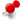Understanding float and doubleClassicListThreaded6 messagesOpen this post in threaded view
|

Understanding float and double

 I am trying to rebuild the Mandelbrot example. I now have a very simple program that creates a mandelbrot fractal. I compare it with a simple (serial) and parallel (threaded) implementation I use forbenchmark results. The results are really great: my serial implementation runs in 2229ms, parallel: 432 ms and openCL in 9ms. An improvement with almost a factor 50! To "dive" deep into a Mandelbrot fractal one needs doubles, else you reach too quickly the resolution of the floats. I noticed that the openCL solution used floats, while my NVidia GTX 1060 and the Intel core i7 920 both have a setting of cl_khr_fp64 = true. The Mandelbrot.cl kernel has a neat way of dealing with floats, making it dependent on the floating point setting. I set it explicitly to double but that did not help. I have listed the kernel below. In my java program I exclusive use double. Anyone any idea how to have the kernel using double variables? #ifdef DOUBLE_FP     #ifdef AMD_FP         #pragma OPENCL EXTENSION cl_amd_fp64 : enable     #else         #pragma OPENCL EXTENSION cl_khr_fp64 : enable     #endif     typedef double varfloat; #else     typedef float varfloat; #endif /**  * For a description of this algorithm please refer to  * http://en.wikipedia.org/wiki/Mandelbrot_set * @author Michael Bien  */ kernel void Mandelbrot     (         const int width,                 const int height,         const int maxIterations,         const double x0,               const double y0,         const double stepX,           const double stepY,         global int *output       ) {     unsigned int ix = get_global_id (0);     unsigned int iy = get_global_id (1);     double r = x0 + ix * stepX;     double i = y0 + iy * stepY;     double x = 0;     double y = 0;     double magnitudeSquared = 0;     int iteration = 0;     while (magnitudeSquared < 4 && iteration < maxIterations)     {         varfloat x2 = x*x;         varfloat y2 = y*y;         y = 2 * x * y + i;         x = x2 - y2 + r;         magnitudeSquared = x2+y2;         iteration++;     }     output [iy * width + ix] = iteration; }
Open this post in threaded view
|

Re: Understanding float and double

 Administrator Hmm, not sure why that doesn't work. Are you really passing doubles into the kernel when you invoke it? If you were still passing floats as arguments, it would give the same results even with doubles used inside the kernel.
Open this post in threaded view
|

Re: Understanding float and double

Open this post in threaded view
|

Re: Understanding float and double

 Administrator Are you setting "-D DOUBLE_FP" when you build the kernel? If not, it would show the behavior you describe.